Email Us: info@lupinepublishers.comCall Us: +1 (914) 407-6109   57 West 57th Street, 3rd floor, New York - NY 10019, USA

# Lupine Publishers Group

## Lupine Publishers

Submit Manuscript

ISSN: 2637-4676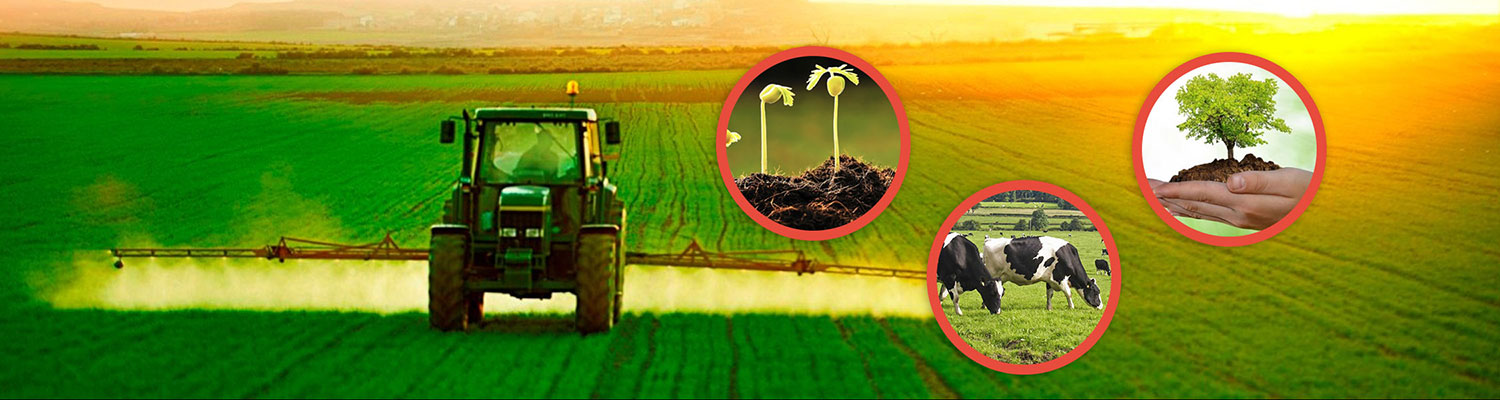# Study of Parameters and Reliability of the Minortress Irrigation System for Work in the Conditions of Mining Farming in Azerbaijan Volume 1 - Issue 4

Rae ZH Aliyev*

• Institute of Erosion and Irrigation of the Azerbaijan Republic, Russia

Received: March 14,2018;   Published: March 23,2018

Corresponding author: Rae ZH Aliyev, Institute of Erosion and Irrigation of the Azerbaijan Republic, Russia

DOI: 10.32474/CIACR.2018.01.000120

## Introduction

In recent years both in the republic, and in the CIS and far abroad, the development of stationary systems of low-intensity irrigation, consisting of micro-irrigation, pulse sprinkling of self-oscillating action, aerosol irrigation, combined micro humidification, drip, pulse-drop and others. This is due to the fact that the system of low- intensity irrigation has a number of significant advantages over other methods of irrigation. Especially promising is the creation of automated sprinkling systems with pulsed apparatuses, microincendiaries of the combined, step-type micro-idlers of the rocker type, pulse-dropping devices of self-oscillating action, etc. It should be noted that these systems can reduce the capital costs for their construction, also provide the principle of both "sprinkling” and "drip irrigation” with daily water consumption of plants. That is, to create optimal conditions for the growth and development of plants in the period of their vegetation.

## Course of the Study

Automated irrigation of crops is the basis of high yields and increase of labour productivity. Consequently, reliable and uninterrupted operation is required from the elements and devices of the automation used. Therefore, from the stage of development of these devices and ending with production and operation, issues of reliability, since the reliability problem is not only a technical, but also an important economic task. The reliability theory developed in the last decades opens great opportunities for a qualitative assessment of the reliability of the existing irrigation technique, incl. systems of micro-irrigation. Reliability is the property of the equipment or system to perform the specified functions, maintain its operational performance for the required period of time or the required operating time. From the existing irrigation technique, a stationary sprinkler system consisting of a large number of similar elements located in a certain order on the field and necessary for irrigation of crops. Determining the reliability of one of these elements solves the problem of reliability of systems. The reliability of stationary irrigation systems in particular equipped with many scientists in the former Soviet Union and abroad has been working on sprinkling systems [1,2]. To study the elements of the calculation of the reliability of low-intensity sprinkler systems (using the example of a pulsed sprinkling of an auto oscillatory action), a technological and feasibility study of the sprinkler systems should be considered. Optimal parameters of the low-intensity irrigation system were determined by.com by searching for the minimum of the function of the specific reduced costs Court. which depends on the area of the system S; the relations of the sides 0; system Q costs; pressure H, corresponding to the pressure of the dictating point; the conditional irrigation period Tu, equal to where, M is the irrigation norm, m; q/is the maximum ordinate of the hydromodule m/s).

Numbers of distribution pipelines N; the distance between irrigation equipment (sprinklers) l; coefficient X, equal to the arrangement of apparatuses by a square of 0.2; the length of the i-th pipeline of the system li of its diameter Di and the flow rate Qi, the coefficients k, m, β depending on the roughness of the inner surface of the pipes; the cost of irrigation equipment (sprinklers, etc). Cg and the blowing node SN; cost of one kWh of electricity;ψ the efficiency of the pump aggregate η, equal to 0.7 of the coefficient of uneven flow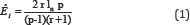where, r is the ratio of the start pressure of the splash P2 to the end pressure of the splash P1) of 1.02 to 1.25; coefficient E0=E1+E2 (E1, E2-normative coefficients of efficiency of capital investments and annual deductions for depreciation and repairs); coefficients b, d depending on the material and the cost of laying pipes and maintenance costs for the maintenance of the personnel of the system En: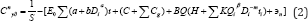Studies have shown that the most economical option is the simultaneous operation of all sprinklers of the system, i.e. when the system performs the limiting dispersal of the irrigation current . The function (2) for this case has a minimum at the points determined by the expressions: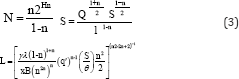Where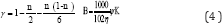Substituting into the formulas (3) and (4) the coefficients corresponding to the steel pipelines (d=1.4, m=5.1, β=1.8, K=0.00107, B=50, E0=0.2; Kh=1,1), we obtain calculation formulas for the determination and which are represented by nomograms (Figure 1) for the convenience of their use. Algorithmic nomograms have been built for pipes made of plastics and other materials. Optimal diameters of pipelines Dopt, I, the number of irrigational pipelines X suspended to the distribution pipeline, the number of sprinklers on the irrigation pipeline y is determined by the followingrelationships: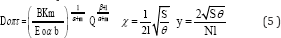When the initial parameters S, q/,θ, M) are changed within the limits of:

30<=S<=300 ra, 0,3 10-7<=q/<=1,0 x 10-7m/c,

O,25<=0<=4, 0,3<=M<=1,0m

1000 m3/ha, the optimal parameters of the system vary within the limits of: 35<=1<=135m; 3<=N<=65; 1<=x<=50; and 1<=y<=33.

Adhering to the areas fixed to specific natural and economic conditions, we define the parameters of low-intensity irrigation systems using the example of impulse sprinkling.

We believe that these areas have been established and are equal to 150-400 hectares and are characterized on the average by the optimal ratio of sides 1-3, and the natural and economic conditions by the irrigation norm 0.35-0.75m and the maximum ordinate of the hydromodule 0.6x10-7-1,0x10-7 m/s. The averaged initial data S=200ha, 0=2; M=0,55m, q/=0,8x10-7m/s, the following optimal parameters of the system correspond: l=50m, N=22, x=10, y=8 obtained using the previously given program and formulas (4.5). For other cultivated crops and natural and economic conditions,these parameters are defined similarly. The obtained values of N, l, x, y, Dopt. I - allow to determine the main design and technological parameters of irrigation equipment (impulse sprinklers of self- oscillating devices): as the head H2, corresponding to the pressure of the beginning of the splash P2, the head H1 corresponding to the pressure of the end of the splash P1; the constructive volume of the pneumo hydro accumulator W0 and the diameter of the nozzle D.

Based on the materials of a number of works by the scientists of the World, and the results of the studies conducted at the Institute of Erosion and Irrigation of the National Academy of Sciences devoted to the definition of certain parameters of irrigation equipment including conventional sprinklers, pulsed action devices, for which we propose the following characteristic controls for determining the main parameters of pulse devices in relation to the head . But, corresponding to the initial pressure in the pneumatic accumulator P0 and at the corresponding atmospheric pressure Pa, the crosssectional areas of the supply line «2, the inlet flow factors μ1 and the nozzle μ2, the resources of the pneumo hydraulic accumulator T *, the sprinkler N*, the coefficients depending on the cost of the sprinkler d1 and d2,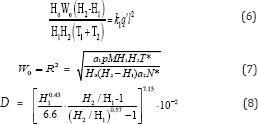It should be noted that equation (6) correlates water supply and water consumption of plants, and equations (7) and (8) characterize, respectively, the optimal sizes of sprinklers and agro technical requirements and rain qualities (the number of sprinkled drops with a diameter of more than 1.0-1.5mm in the total flow of the sprinkler should be less than 10%).

To each group M, q, S, θ there correspond quite definite values of x, y, N, l parameters of the system and H2 H1, W0, D parameters of the impulse device. Taking into account that on the real system T1=>T2 and the sum of the hydraulic resistances to the worst by the filling conditions of the pneumo hydro accumulator of the system , equation (6) can be represented in the form: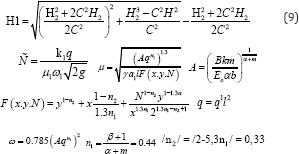Qi is a coefficient equal to 2.64 for steel pipes. 10-3/Equations (7.8 and 9) together with the formula of AP Rousetskii are a closed system of equations.

Figure 1 below shows the graphical solution of this system. Knowing the economic range of the impulse device and the parameter C of the system (C=3-5), the search for a solution should be carried out according to the corresponding curve C in the following order in a1, a4, a7, a10 in C=3, a2, a5, a8, a11 for C=4 and a3, a6, a9, a12 for C=5. The parameters of the sprinkler atintermediate values of C are determined by interpolation (Figure 1).

Figure 1: Nomogram for determining the parameters of a pulsed apparatus.Using the results obtained earlier, we determine the design and technological parameters of the impulse device for the same natural and economic conditions . When the apparatus is arranged in squares, its effective range is R=21-35, and the parameter C=3. With the help of the nomogram (Figure 1) and the input quantities K and C, the following parameters of the pulsed apparatus were obtained:

P1 = 450kPa, P2 = 700kPa, W0 = 0.19m3, D = 20mm

To solve a set of measures for the reliability of systems is the reliability of the elements of sprinkler systems. Researches of indicators of reliability of basic elements of stationary sprinkling systems are resulted results of theoretical and experimental researches, noise immunity of devices of remote control is estimated, parameters of dispersion of the irrigation norms dropped out by separate sprinklers are established and kinds and parameters of distributions of working out before failure of the basic elements of systems of sprinkling are established. At the time when the remote control device receives the "object selection” command, transient processes appear in the network of process pipelines. It is established that the pressure P can take values below the statistical adjustment pressure of the remote control device P/0 and the duration of the false signal exposure can reach 0.15-1.2 seconds.

The following experimentally confirmed relationship between the processing time of the command signal tk, the stroke of the rod h with the effective area of the membrane Fe, the cross-sectional area of the tube connecting the process pipeline to the working cavity «, the coefficient of rigidity of the return spring K2, the specific gravity of water yb and the acceleration of gravity g: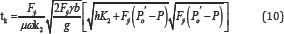According to the formula (10), the time of reconfiguration of the automated telecontrol device was estimated with instant decrease. Pressure in the cavity of the hydraulic drive to atmospheric pressure of 340 kPa, corresponding to the pressure "object selection”, 0.34 is required, which is 1.5 times longer than the duration of the false signal .

Dependencies (10) allow not only to estimate the time for reconfiguration of the automatic control system of the irrigation technological process via the telecontrol system channel, but also to assign its design value to the requirements of noise immunity of the elements of the telecontrol systems. Dispersion of the pressure of the beginning of the splash of pulsed self-oscillating devices leads to the dispersion of their volumes of splash ΔW, hence, to the rain layer M, since they are interconnected by dependences(The number of cycles of the device in the year and the area of its maintenance). Tests of pulsed devices (sample 100) and subsequent processing of the obtained data showed that the distributions of P2 and P1 are subject to the normal law with coefficients of variation vp2=0.04 and vp1=0.093. I mean,

What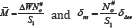The distribution density M can be represented as: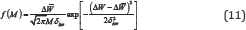The distribution (11) can be used to estimate the damage from the structural imperfection of impulse devices. Obviously, the smaller the ratio, the better the apparatus. Investigations (Table 1) for determining the types and parameters of distributions of developments to failure (,σ - mean and squared deviation) of typical devices of sprinkler systems, including the most common elements in hydro automatics: underground retractable hydrants. (Elements 1,2), devices of automated telecontrol systems (Elements 3, 4), Table 1.rotary mechanisms (and element 5) as well as impulse sprinklers (elements 6,7, 8, 9). It is established that the distribution of the recovery time of these elements can be characterized by the mean recovery time Tg.

Table 1: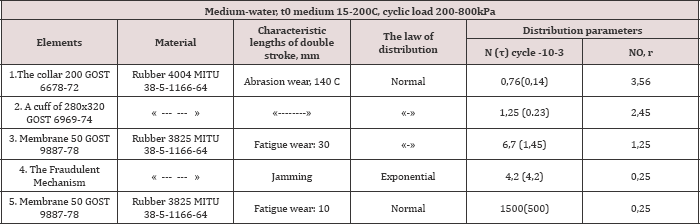## Discussion of Research Results

As a result of special studies, the following relationship was obtained between the average life of parts made of rubber or plastics Tcp, the average number of cycles N#0 per year and the experimental value of the aging parameter of these materials in water T0 equal to 6.8 years: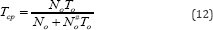In addition (Table 1) to these measures, it is necessary to develop organizational measures to solve the optimal level of operational reliability of stationary systems. For organizational events, the implementation of preventive works and the right timing are of particular importance. Determining the timing of prevention is one of the main problems of preventive maintenance, closely related to the content of preventive work and the organization of their implementation . These terms are usually determined by research the following objective function: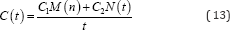where, C1, C2, respectively, the cost of replacing parts when eliminating the failure and preventive replacements; M(t), N(t)- respectively, the average number of replacements in case of failure and preventive maintenance in time t.

## Scientific Conclusion

The exponent of the evaluation of perfection made by.com is expedient for applying with the purpose of a choice of the most perfect design, as it economically generalizes parameters of maintainability, maintainability and safety of sprinkler systems.

Close## Online Submission System

### Submit ManuscriptClick here for Manuscript Sample Templates### Drag and drop files here

or

( For multiple files submission, zip them in a single file to submit. For file zipping software Download )